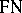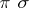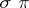# Alternative set theory

(diff) ← Older revision | Latest revision (diff) | Newer revision → (diff)

A theory created as an answer to the question that should be asked by every philosophically thinking mathematician: How is it possible that certain results of infinitary mathematics are applicable in the real world, while results other are not?

Present mathematics is founded on the classical Cantor theory of actually infinite sets (cf. Set theory). Nowadays however (1996), the existence of such sets in the real world is, to say the least, dubious. Even if one admits that such sets do exist in the real world, they are not those which infinite mathematics applies its results to.

The results of infinite mathematics are applied to certain phenomena that accompany the appearance of large sets. Here, a "large set" is meant to be a set that is finite from the classical point of view but is not located, as a whole and along with each of its elements, in front of the horizon currently limiting the view of the given set. Here, "view" is understood in much more than just a visual sense, essentially as a grasping and holding of some field of phenomena.

If infinity is applicable to certain phenomena which show themselves on large sets, then it has to be already present there in some form. This form of infinity is called natural infinity. Alternative set theory is a theory of natural infinity. It works with objects called classes that are further classified as sets and semi-sets. Sets are definite, sharply defined, unchangeable from the classical point of view. Semi-sets are their unsharp parts. Above all, it is the semi-sets that represent the phenomena which appear when viewing large sets and to which the results of infinitary mathematics are applied.

A set is naturally finite if all its subclasses are sets. Every semi-set, as well as every set containing a semi-set, is naturally infinite. Finiteness and infiniteness hence depend on the point view and on the place of the corresponding horizon. Static alternative set theory studies the phenomenon of natural infinity under a given fixed point of view. Dynamical alternative set theory, on the other hand, considers a changing point of view and a moving horizon.

The classof all natural numbers that lie in front of the horizon of a considered view is a semi-set. The classes that can be enumerated by numbers fromare called countable. The classbehaves similarly as the classical set of natural numbers. However, results about numbers when applied are less evident as one approaches the horizon.

Classes are extensions of concepts and there are different sorts of classes corresponding to different sorts of concepts.

Positively-defined concepts are represented by so-called-classes, which are countable unions of sets. (As an example of such a concept, the colour red is first learned on basic sharp shades of red, then on less distinct shades, etc., to the horizon.) Negatively-defined classes are represented by-classes, which are countable intersections of sets. It can be proved that every class that is at the same timeandmust be a set. In other words, if a set contains two disjoint semi-sets representing positively-defined concepts, then there must be an element of the set that is not contained in either of the semi-sets. More complicated concepts are represented by-classes,-classes, etc.; this hierarchy has similar properties as the Borel hierarchy of sets in classical topology (cf. also Topological structure (topology); Borel system of sets; Borel set of ambiguous class).

So, natural infinity is closely related to vagueness. In that sense alternative set theory is a study of vague collections. It is desirable to find appropriate scales for membership of objects in various types of semi-sets. Different scales may be suitable for different types of semi-sets, some scales may not even be linear; other semi-sets need not even have any suitable scale (all their element belong vaguely to the semi-sets).

Indiscernibility equivalences present an important example of-classes. If an infinite set is completely located in front of the horizon, then its elements make up monads of mutually indiscernible elements. These monads are-classes and their configuration creates a topological shape of the set. One can then identify only so-called figures, that is, those parts of the set that with each point contain also its monad. It can be proved that such topological shapes correspond to separable metric spaces (cf. Metric space; Separable space). Similarly, time, motion and generally all sorts of changes of shape in time can be modelled in this way discretely and continuously at the same time.

The interpretation of topological shapes as outer appearances of indiscernibility enables one to consider so-called invisible shapes of sets. For example, an indiscernibility equivalence is created on the class of inhabitants of Europe by certain selected properties. The equivalence then induces a corresponding topological metrizable shape that cannot be observed from inside.

Classes that are figures in an equivalence of indiscernibility are called real. These are exactly the classes that are identifiable in the real world. On the other hand, imaginary classes are the classes that are only conceivable. However, being conceivable is a vague concept, somewhere between pure consistence and absurdity. In addition, two imaginary classes may exclude each other. By deciding for one of them a certain branch of alternative set theory is entered.

The phenomenological analysis of classical infinity draws the conclusion that this infinity is just the sharply interpreted natural infinity with a number of imaginary classes. From this point of view, Cantor's set theory is only a branch (or possibly several different branches) of alternative set theory.